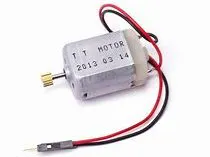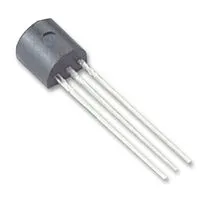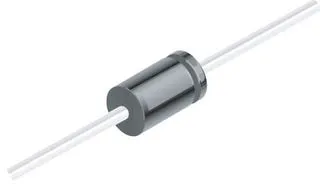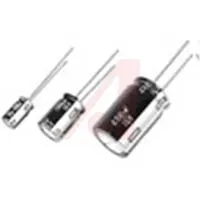# How to Use Motor

Designed for beginners, this project will show you how to control a motor by doing accelerate and decelerate.

• 595 views
• 14 respects

## Components and suppliesArduino UNO & Genuino UNO
×1Jumper wires (generic)
×1Resistor 10k ohm
×1General Purpose Transistor NPN
×11N4007 – High Voltage, High Current Rated Diode
×1×1
×1
 DC motor (generic)
×1Capacitor 220 µF
×1

## Apps and online services

DC Motors can operate in both directions, depending on how the current is subjected to it.

Benefits:

• Not expensive,
• Easy to use (2 wire),
• works with a continuous voltage,
• can achieve very high rotation speeds.

• Few torque (car high speed, for + torque = > gear motor),
• Speed control only (no possibility of controlling the axis position),
• relatively costly in current,

### So I will speak about a code example :

`````` void setup() {
pinMode(13, OUTPUT);
}
void loop() {
digitalWrite(13, HIGH);

}
``````

In this code there are 2 fonction vital to the operation of the code.

Without them the code would not be importable in the Arduino card.

The 2 fonctions are :

`````` void setup() {
}
``````

And :

`````` void loop() {
}
``````

When the program needs to be realized, the setup function will initialize the ports ( from 1 to 13 for digital enter ) and the type of action [ OUTPUT ( power output ) or INPUT ( power input ) ] that the program asks.

The loop function will acomplish the code to infinity and LOOP ( It's very important for the rest ).

### Exemple : If I setup this code in my card

`````` void setup() {
pinMode(13, OUTPUT);
pinMode(5, OUTPUT);
}
void loop() {
digitalWrite(13, HIGH);
digitalWrite(5, HIGH);
}
``````

In this code the function " void setup " will initialize the ports ( 13 and 5 ) and the type of action ( OUTPUT ) that the program asks.

After, he'll do the program over and over ( your LED will not blink ).

If you want to slow down the program you must set that in your code :

``````delay(1000);
``````

The program will be stop for 1000 milliseconds ( 1 second ). But you are required to say how long you stop it in milliseconds.

## Code

##### Motor Arduino
```int motorPin = 9;  // define the pin the motor is connected to
// (if you use pin 9,10,11 or 3you can also control speed)

/*
* setup() - this function runs once when you turn your Arduino on
* We set the motors pin to be an output (turning the pin high (+5v) or low (ground) (-))
* rather than an input (checking whether a pin is high or low)
*/
void setup()
{
pinMode(motorPin, OUTPUT);
}

/*
* loop() - this function will start after setup finishes and then repeat
* we call a function called motorOnThenOff()
*/

void loop()                     // run over and over again
{
motorOnThenOff();
//motorOnThenOffWithSpeed();
//motorAcceleration();
}

/*
* motorOnThenOff() - turns motor on then off
* (notice this code is identical to the code we used for
* the blinking LED)
*/
void motorOnThenOff(){
int onTime = 2500;  //the number of milliseconds for the motor to turn on for
int offTime = 1000; //the number of milliseconds for the motor to turn off for

digitalWrite(motorPin, HIGH); // turns the motor On
delay(onTime);                // waits for onTime milliseconds
digitalWrite(motorPin, LOW);  // turns the motor Off
delay(offTime);               // waits for offTime milliseconds
}

/*
* motorOnThenOffWithSpeed() - turns motor on then off but uses speed values as well
* (notice this code is identical to the code we used for
* the blinking LED)
*/
void motorOnThenOffWithSpeed(){

int onSpeed = 200;  // a number between 0 (stopped) and 255 (full speed)
int onTime = 2500;  //the number of milliseconds for the motor to turn on for

int offSpeed = 50;  // a number between 0 (stopped) and 255 (full speed)
int offTime = 1000; //the number of milliseconds for the motor to turn off for

analogWrite(motorPin, onSpeed);   // turns the motor On
delay(onTime);                    // waits for onTime milliseconds
analogWrite(motorPin, offSpeed);  // turns the motor Off
delay(offTime);                   // waits for offTime milliseconds
}

/*
* motorAcceleration() - accelerates the motor to full speed then
* back down to zero
*/
void motorAcceleration(){
int delayTime = 50; //milliseconds between each speed step

//Accelerates the motor
for(int i = 0; i < 256; i++){ //goes through each speed from 0 to 255
analogWrite(motorPin, i);   //sets the new speed
delay(delayTime);           // waits for delayTime milliseconds
}

//Decelerates the motor
for(int i = 255; i >= 0; i--){ //goes through each speed from 255 to 0
analogWrite(motorPin, i);   //sets the new speed
delay(delayTime);           // waits for delayTime milliseconds
}
}
```

## Schematics

motor_HsaXQNAJyF.fzz

• 8,829 views
• 37 respects

#### How to Use a L293D Chip with Arduino and a Motor

Project tutorial by Austin Detzel

• 9,068 views
• 14 respects

• 18,249 views
• 48 respects

• 16,616 views
• 24 respects

by Jasleen

• 8,274 views